This post will guide you how to use Google Sheets YEARFRAC function with syntax and examples.

## Description

The Google Sheets YEARFRAC function returns the number of years between two dates including fractional years. And you can use this function to calculate age with a birthdate.

The YEARFRAC function can be used to get a decimal value that represents fractional years between two given dates in google sheets. The purpose of this function is to get the fraction of a year between two dates and its returned value is a decimal number.

The YEARFRAC function is a build-in function in Google Sheets and it is categorized as a Date function.

## Syntax

The syntax of the YEARFRAC function is as below:

=YEARFRAC(start_date, end_date, [day_count_convention])

Where the YEARFRAC function arguments are:

• Start_dateThis is a required argument. The starting date from which you want to count the number of years. It must be a cell reference that containing a date, or a function that returning a date type or a number.
• End_date – This is a required argument. The end date that is considering in the calculation. It must be a cell reference that containing a date, or a function that returning a date type or a number.
• day_count_convention This is an optional argument.an type of what day count method to use.
 type calculation 0(default) 30/360 1 Actual/actual 2 Actual/360 3 Actual/365 4 30/360

Note:

• this function is mostly used in a financial setting.
• You need to make sure that the inputs to the function are either cell reference that containing valid dates.

## Google Sheets YEARFRAC Function Examples

The below examples will show you how to use google sheets YEARFRAC Function to return the number of years between two dates.

1# a start date is in Cell A1, and an end date is in Cell B1, and using the YEARFRAC function to count the years between two dates:

`=YEARFRAC(A1,B1)`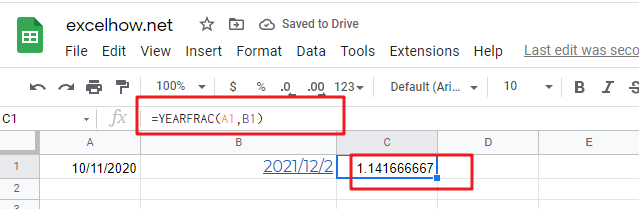2# if you want to gt a whole number , and you can use the INT function in combination with the YEARFRAC function, type:

`=INT(YEARFRAC(A1,B1)`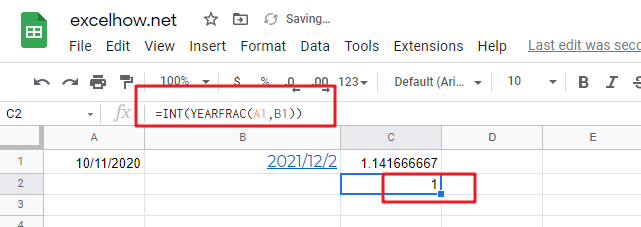3# if you want to get the current age based on a birthdate in Cell A2, and you can use the following formula based on the INT function, the TODAY function and the YEARFRAC function, type:

`=INT(YEARFRAC(A2,TODAY()))`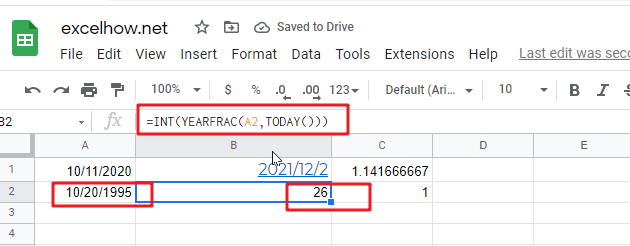This post will guide you how to use Google Sheets YEAR function with syntax and examples.

## Description

The Google Sheets YEAR function returns a four-digit year from a given date value, the year is returned as an integer ranging from 1900 to 9999. So you can use the YEAR function to get the year component of a date. You can also use this function to extract the year portion from an Excel date and feed the returned value into another formula.

The YEAR function can be used to get the year component of a data as a 4-digit number in google sheets. The purpose of this function is to get the yar from a data and the returned value is a year as 4-digit number.

The YEAR function is a build-in function in Google Sheets and it is categorized as a Date function.

## Syntax

The syntax of the YEAR function is as below:

=YEAR (serial_number)

Where the YEAR function arguments is:

• Serial_number -This is a required argument. A valid date value that contains the year component you want to extract. Dates should be entered by using the DATE function, or as results of other formulas or functions. For example, use DATE(2019,3,15) for the 15th day of March, 2019.

Note:

• A serial date is how the google sheets stores dates and it represents the number of days since 1900-01-01, so the January 1, 1900 date is serial number 1 by default.
• If the serial_number is not a valid date, it will return #VALUE! Error.

## Google Sheets YEAR Function Examples

The below examples will show you how to use google sheets YEAR Function to return an integer number that representing the year component of a given date.

1# get year of the date in Cell A1, enter the following formula in Cell B1.

`=YEAR(A1)`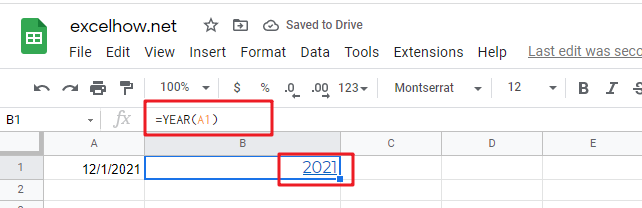This post will guide you how to use Google Sheets WORKDAY.INTL function with syntax and examples.

## Description

The Google Sheets WORKDAY.INTL function returns the serial number of the date before or after a specified number of workdays. And this function allows you to exclude weekends and holidays as non-working days. You can use the WORKDAY.INTL function to calculate working days excluding weekdays and holidays.

The WORKDAY.INTL function can be used to calculate the date after a specified number of workdays excluding specified weekend days and in google sheets. The purpose of this function is to get date n working days in future or past.

The WORKDAY.INTL function is a build-in function in Google Sheets and it is categorized as a Date function.

## Syntax

The syntax of the WORKDAY.INTL function is as below:

= WORKDAY.INTL (start_date, days, [weekend], [holidays])

Where the WORKDAY.INTL function arguments is:

• Start_dateThis is a required argument. The starting date from which you want to count the number of working days. The date should be typed as a valid Excel time a serial date.
• days – This is a required argument. The number of working days that you want to add. A positive value for days yields a future date; a negative value yields a past date.
• Weekend This is an optional argument. Determine which days of the week that are weekend days and are not considered working days. Weekend is a weekend number and it can be one of the following numbers.
 weekend-number Weekend days 1 or omitted Saturday, Sunday 2 Sunday, Monday 3 Monday, Tuesday 4 Tuesday, Wednesday 5 Wednesday, Thursday 6 Thursday, Friday 7 Friday, Saturday 11 Sunday only 12 Monday only 13 Tuesday only 14 Wednesday only 15 Thursday only 16 Friday only 17 Saturday only
• holidays – This is an optional argument. The list of holidays that you want to exclude from the working days. It can be a range of cells that contain the holiday dates or it can be a list of serial numbers that represent the holiday dates.

Note:

• If any argument is not a valid date, a #VALUE! Error is returned.
• If start date value plus days is an invalid date, the WORKDAY function returns #NUM! Error.
• A serial date is how the google sheets stores dates and it represents the number of days since 1900-01-01, so the January 1, 1900 date is serial number 1 by default.
• If days value is not an integer number, it will be truncated.
• If a weekend string is of invalid length or contains invalid characters, WORKDAY.INTL returns the #VALUE! Error value.

## Google Sheets WORKDAY.INTL Function Examples

The below examples will show you how to use google sheets WORKDAY.INTL Function to return the working days from the start date.

1# get the date 50 WORKDAY.INTLs from the starting date “3/20/2021”, enter the following formula in Cell B1.

=WORKDAY.INTL(DATE(2021,3,20),50)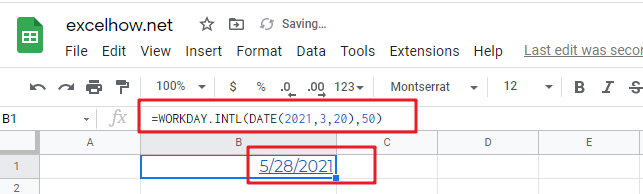This post will guide you how to use Google Sheets WEEKNUM function with syntax and examples.

## Description

The Google Sheets WEEKNUM function returns the week number of a specific date, and the returned value is ranging from 1 to 53. And this function will start to count the week number from January 1 and the Sunday is the first day in one week by default.

The WEEKNUM function can be used to get a number representing the week of the year where the provided date falls in google sheets. The purpose of this function is to get the week number for a given date and it will return a number between 1 and 54.

The WEEKNUM function is a build-in function in Google Sheets and it is categorized as a Date function.

## Syntax

The syntax of the WEEKNUM function is as below:

=WEEKNUM (serial_number,[return_type])

Where the WEEKNUM function arguments is:

• Serial_numberThis is a required argument. A date that you want to get the week number. The dates should be typed by the DATE function to make sure it is a valid date. For example, use DATE(2021,3,20) to pass into the WEEKNUM function and then it will return the week number.
• return_typeThis is an optional argument. A number that determines the first day of the week for the year, the default value is 1.
 Return_type Week begins on System 1 or omitted Sunday 1 2 Monday 1 11 Monday 1 12 Tuesday 1 13 Wednesday 1 14 Thursday 1 15 Friday 1 16 Saturday 1 17 Sunday 1 21 Monday 2

Note:

• If serial_number is out of range for the current date base value, a #NUM! Error is returned.
• If return_type is out of the range specified in the table above, a #NUM! Error is returned.
• A serial date is how the google sheets stores dates and it represents the number of days since 1900-01-01, so the January 1, 1900 date is serial number 1 by default.

## Google Sheets WEEKNUM Function Examples

The below examples will show you how to use google sheets WEEKNUM Function to return the week number from a date.

1# get number of the week from a date value 2021/03/20, enter the following formula in Cell B1.

`=WEEKNUM(DATE(2021,3,2018))`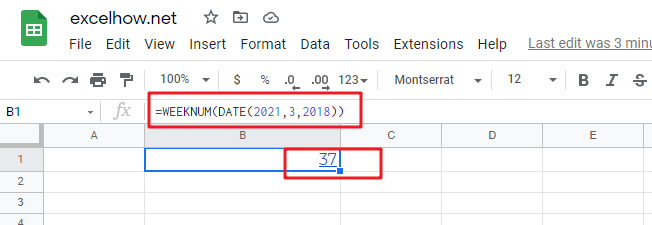This post will guide you how to use Google Sheets WEEKDAY function with syntax and examples.

## Description

The Google Sheets WEEKDAY function returns an integer value representing the day of the week for a given date and the value is range from 1 to 7. You can use this function to get the weekday number for a supplied date. and it will return 1 for Sunday and returns 7 for Saturday. you also can use the WEEKDAY function to combine with other formulas or functions.

The WEEKDAY function can be used to get a number representing the day of the week of the date provided in google sheets. The purpose of this function is to get the day of the week as a number and it will return a number between 0 and 7.

The WEEKDAY function is a build-in function in Google Sheets and it is categorized as a Date function.

## Syntax

The syntax of the WEEKDAY function is as below:

=WEEKDAY (serial_number,[return_type])

Where the WEEKDAY function arguments are:

• Serial_numberThis is a required argument. A date that you want to get the weekday of. the dates should be typed by the DATE function to make sure it is a valid date. For example, use DATE(2018,3,20) to pass into the WEEKDAY function and then it will return the weekday of this date. It must be a reference to a cell that containing a date or a date serial number of the type returned by the N function.
• return_type – This is an optional argument. A number that determines the type of return value.
 Return_type Number returned 1 or omitted Numbers 1 (Sunday) through 7 (Saturday). Behaves like previous versions of Microsoft Excel. 2 Numbers 1 (Monday) through 7 (Sunday). 3 Numbers 0 (Monday) through 6 (Sunday). 11 Numbers 1 (Monday) through 7 (Sunday). 12 Numbers 1 (Tuesday) through 7 (Monday). 13 Numbers 1 (Wednesday) through 7 (Tuesday). 14 Numbers 1 (Thursday) through 7 (Wednesday). 15 Numbers 1 (Friday) through 7 (Thursday). 16 Numbers 1 (Saturday) through 7 (Friday). 17 Numbers 1 (Sunday) through 7 (Saturday).

Note:

• If serial_number is out of range for the current date base value, a #NUM! error is returned.
• If return_type is out of the range specified in the table above, a #NUM! error is returned.
• A serial date is how the Microsoft Excel stores dates and it represents the number of days since 1900-01-01, so the January 1, 1900 date is serial number 1 by default.

## Google Sheets WEEKDAY Function Examples

The below examples will show you how to use google sheets WEEKDAY Function to return a weekday of a date.

1# get day of the week with the default return type 1, enter the following formula in Cell B1.

`=WEEKDAY(DATE(2021,11,25))`

it means that the weekday of that date is Tuesday.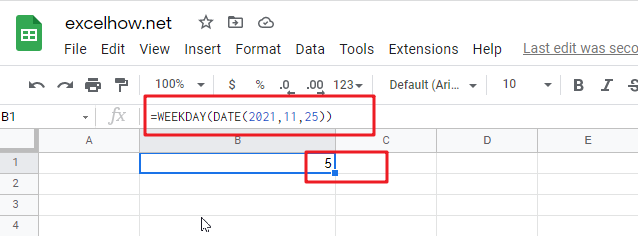This post will guide you how to use Google Sheets TIMEVALUE function with syntax and examples.

## Description

The Google Sheets TIMEVALUE returns the decimal number of the time represented by a text string. It will convert a time represented by a text string into a time value. and the returned value is range from 0 to 0.99988, it indicated the times from 0:00:00 to 23:59:59.

For example, when you fed the text string “7:13 PM” into TIMEVALUE function, and it returns 0.80069. the returned value is a numeric value and this value can be used to manipulate with another formulas.

The TIMEVALUE function can be used to get the fraction of a 24-hour day the time represents. The purpose of this function is to get a valid time from a text string and its returned values is a valid time as a decimal number.

The TIMEVALUE function is a build-in function in Google Sheets and it is categorized as a Date function.

## Syntax

The syntax of the TIMEVALUE function is as below:

=TIMEVALUE (time_text)

Where the TIMEVALUE function arguments is:

• time_text -This is a required argument. A text string that represents a time, within the text string, the hours, minutes and seconds components should be separated by colon characters.

Note:

• the date information in text string will be ignored.
• if the time_text is not a valid time in a text format , it will return #VALUE! error.

## Google Sheets TIMEVALUE Function Examples

The below examples will show you how to use Google sheets TIMEVALUE Function to convert a text string that representing a time in Excel.

1# get decimal part of a day, with only the time portion specified, enter the following formula in Cell B1.

`=TIMEVALUE("7:27 PM")`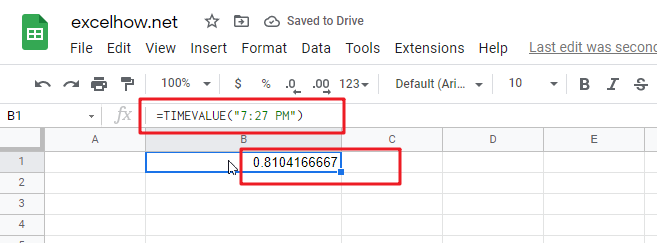2# get decimal part of a day, with date and time specified, enter the following formula in Cell B3.

`=TIMEVALUE("19-March-2018 7:28PM")`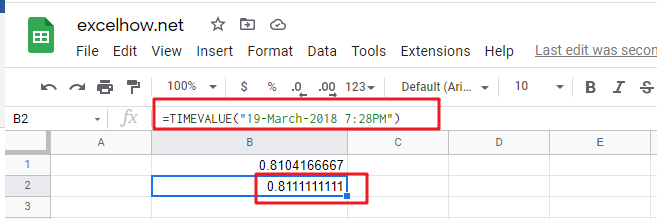This post will guide you how to use Google Sheets TODAY function with syntax and examples.

## Description

The Google Sheets TODAY function get the current date as a date value. And it will be updated each time when your worksheet is changed or opened. This function does not need to add any arguments.

You can use the TODAY function to get the current date displayed on your worksheet. And it also can be used for calculating intervals. For example, if you want to know that person’s age (he was born in 1984), you can use the following formula:

=YEAR(TODAY())-1984

This formula will use the TODAY function as an argument for the YEAR function to get the current year, and then subtracts 1984, getting his age.

The TODAY function is a build-in function in Google Sheets and it is categorized as a Date function.

## Syntax

The syntax of the TODAY function is as below:

=TODAY()

There are no any arguments for the TODAY function, but you still need to use empty parentheses ().

Note:

• TODAY function will be updated on every edit made to the spreadsheet and it will impact spreadsheet performance.
• TODAY function will create a date without the current time, and if you wish to get the current date and time, and you can use the NOW function.
• TODAY function will always get the current date and the last date in the spreadsheet was recalculated, rather than remaining at the date when it was first entered.

## Google Sheets TODAY Function Examples

The below examples will show you how to use google sheets TODAY Function to return the current system date.

1# using the TODAY function to get the current date, enter the following formula in Cell B1.

`=TODAY()`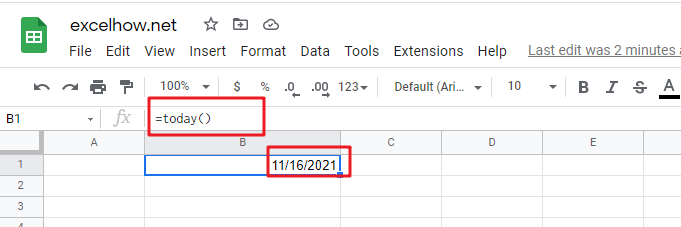2# Get the current date and then subtracts 10 days, using the following formula:

`=TODAY() -10`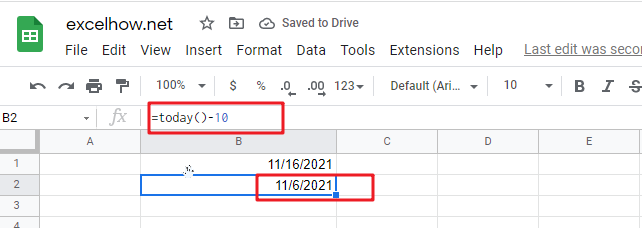3# Get the number of days between the current date and 03/14/2022, type the following formula:

`=DATEVALUE("03/14/2019")-TODAY()`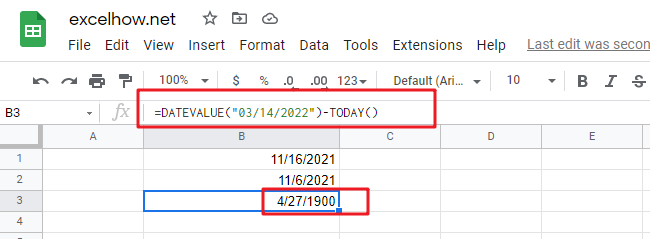4# Get the current day of the month, using the following formula:

`=DAY(TODAY())`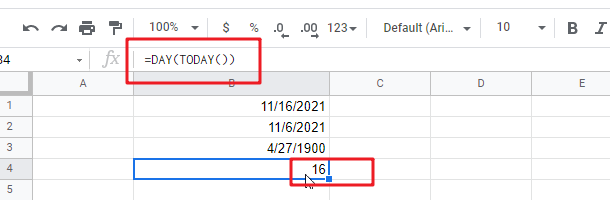5# Get the current month of the year, type the following formula in Cell B1:

`=MONTH(TODAY())`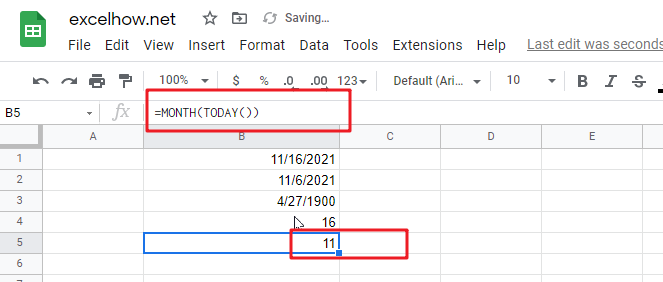This post will guide you how to use Google Sheets TIME function with syntax and examples.

## Description

The Google Sheets TIME function converts an hour, minute and second into a time.

The TIME function can be used to create a time with individual hour, minute and second components in google sheets. The purpose of this function is to create a time with the specified hours, minutes and seconds and it also will return a decimal number representing a particular time in google sheets.

The TIME function is a build-in function in Google Sheets and it is categorized as a Date function.

## Syntax

The syntax of the TIME function is as below:

= TIME(hour, minute, second)

Where the TIME function arguments are:

• Hour -This is a required argument. A number from 0 (zero) to 32767 representing the hour. If the value is greater than 23 and then it will be divided by 24 and the remainder will be treated as the hour value. For example, the TIME(28,0,0) is equal to TIME(4,0,0).
• Minute – This is a required argument. A number from 0 to 32767 representing the minute. If the minute value is greater than 59 and it will be converted to hours and minutes (it means that every 60 minutes will add one hour to hour value). For example, TIME(1,75,0) = TIME(2,15,0).
• Second – This is a required argument. A number from 0 to 32767 representing the second. If the second value is greater than 59 and it will be converted to hours, minutes, and seconds (it means that every 60 seconds will add 1 minute to minute value). For example, TIME(1,20,75) = TIME(1,21,15) .

Note:

• All of arguments must be numbers and if a string or a cell reference that containing a text string is provided, and TIME function returns #VALUE! Error message.
• TIME function will truncate decimal values of input into the function, for example, an hour component value of 10.58 will be recognized as 10.

## Google Sheets TIME Function Examples

The below examples will show you how to use google sheets TIME function to create a decimal number representing a particular time.

#1 =TIME(A2,B2,C2)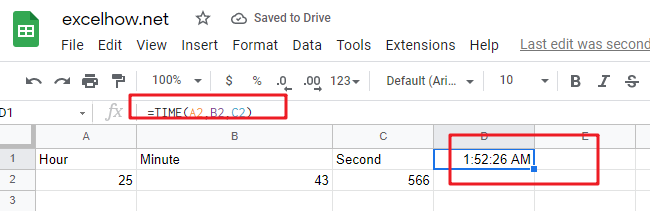This post will guide you how to use Google Sheets SECOND function with syntax and examples.

## Description

The Google Sheets SECOND function returns the seconds of a time value. Or returns an integer value that represent the second component of a given excel time. And the return value is between 0-59.

For example, if you pass a time of 6:32:54 PM into SECOND function, and it will return 54. You can use this function to extract the second component from a time value.

The SECOND function can be used to get the second component of a given time in google sheets. The purpose of this function is to get the second as a number from a time and its returned values is a number that representing time. You can use the SECOND function to extract the second into a cell or you can also pass the result into another function as a new formula.

The SECOND function is a build-in function in Google Sheets and it is categorized as a Date function.

## Syntax

The syntax of the SECOND function is as below:

=SECOND (Time )

Where the SECOND function arguments is:

• Time -This is a required argument. The time from which you want to extract the second component. it may be typed as text string within quotation marks (for example, “13:52 PM”), or it can be a decimal numbers (for example, 0.5), or the result of a formula (for example, TIMEVALUE(“13.54:30 PM”)).  It must be a cell reference that containing a date or time, or a function that returning a date/time type or a number.

Note:

• A serial date is how the google sheets stores dates and times and it represents the number of days since 1900-01-01, so the January 1, 1900 date is serial number 1 by default.
• If the `Time` argument is not a valid time, it will return #VALUE! Error.
• `Time `argument can be supplied to the SECOND function as a time text string or as a decimal number. If you wish to create a time value with separate hour, minute and second, you can use the TIME function.

## Google Sheets SECOND Function Examples

The below examples will show you how to use google sheets SECOND Function to return the seconds of a given time value.

1# get the seconds from an Excel time 6:38:45, enter the following formula in Cell B1.

`=SECOND("6:38:45")`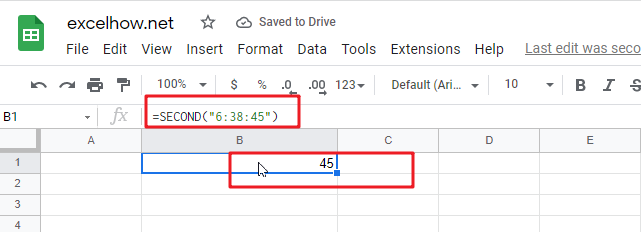2# Get the second portion of the date with no second portion specified, enter the following formula in Cell B3.

`=SECOND("6:38")`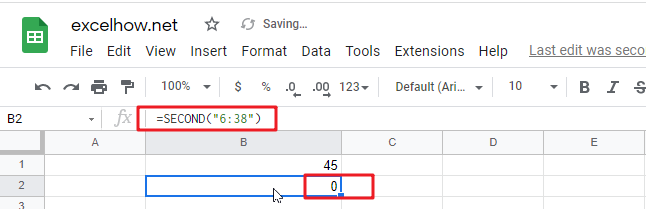or

`=SECOND(TIMEVALUE("6:38 PM"))`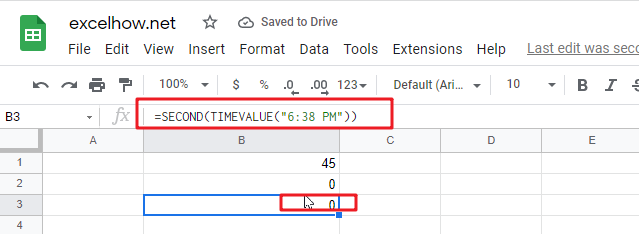It will return 0.

This post will guide you how to use Google Sheets NOW function with syntax and examples.

## Description

The Google Sheets NOW function returns the serial number of the current date and time. And it will be updated each time when your worksheet is changed or opened. This function does not need to add any arguments.

The NOW function can be used to get the current date and time in google sheets. The purpose of this function is to get the current date and time and its returned values is a number that representing the date and time in google sheets.

The NOW function is a build-in function in Google Sheets and it is categorized as a Date function.

## Syntax

The syntax of the NOW function is as below:

=NOW ()

Note:

• A serial date is how the google sheets stores dates and it represents the number of days since 1900-01-01, so the January 1, 1900 date is serial number 1 by default.
• Numbers to the right of the decimal point in the serial number represent the time; numbers to the left represent the date. For example, the serial number 0.5 represents the time 12:00 noon. And the serial number 0.25 represents the time 6:00 AM.
•  If the NOW function does not update cell values when you expect it to, you might need to change settings that control when the sheet recalculates.
• Now function will be updated on every edit made to the spreadsheet and it will impact spreadsheet performance.
• NOW function will provide the current date and time. If you wish to create a date without the current time, using TODAY function.
• NOW function will always get the current date and time and the last time in the spreadsheet was recalculated, rather than remaining at the date and time when it was first entered.
• The date or time component of NOW function can be hidden by changing the number formatting.

## Google Sheets NOW Function Examples

The below examples will show you how to use google sheets NOW Function to return the current date and time.

1# get the current date and time, enter the following formula in Cell B1.

`=NOW()`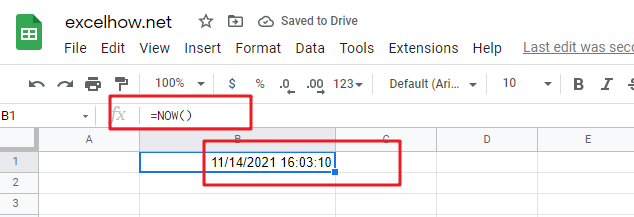2# get the date and time 12 hours ago, you can use the following formula:

`=NOW()-0.5`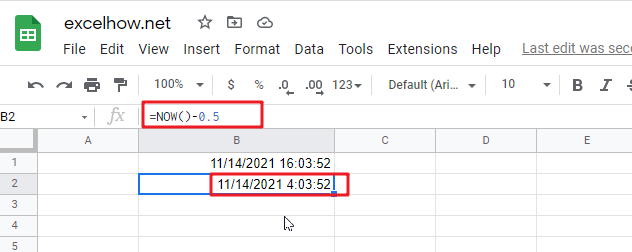3# get the date and time 5 days in the future, just use the following formula:

`=NOW() + 5`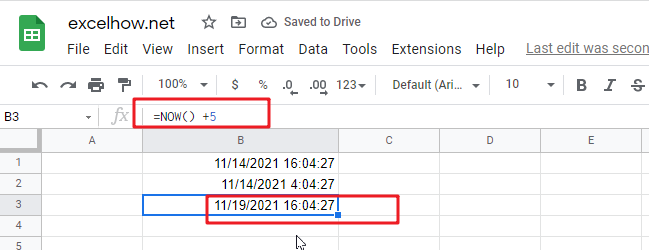This post will guide you how to use Google Sheets NETWORKDAYS.INTL function with syntax and examples.

## Description

The Google Sheets NETWORKDAYS.INTL function returns the number of net working days between tow given days excluding specified weekend days and holidays.

The NETWORKDAYS.INTL function can be used to calculate the number of working days between dates in google sheets. And NETWORKDAYS.INTL will exclude weekends optionally and provides a way to specify which days of the week should be considered as weekends.

The purpose of this function is to get the number of working days between two dates and its returned values is a number that representing days.

This function is more complex that the NETWORKDAYS function because it allows you to control which days of the week are considered weekends.

The NETWORKDAYS.INTL function is a build-in function in Google Sheets and it is categorized as a Date function.

## Syntax

The syntax of the NETWORKDAYS.INTL function is as below:

=NETWORKDAYS.INTL (start_date, end_date, [weekend], [holidays])

Where the NETWORKDAYS.INTL function argument is:

• Start_date -This is a required argument. The starting of date from which to calculate the number of working days.
• End_date – this is a required argument. The ending of date from which to calculate the number of working days.
• Weekend – this is an optional argument. A number that representing which days of the week are considered as weekends.
• Holidays – this is an optional argument. A list of non-working days.

Note:

• `Weekend` argument can be specified using seven 0’s and 1’s, where the first number represents Monday and the last number represents Sunday. A 0’s means that the day is a working day, and a 1’s means that the day is a weekend. For example, “0000011” means that Saturday and Sunday are weekends.
• `Weekend` argument also can be specified as a single number, you can refer to the below table displaying the weekend numbers and their corresponding weekend days.
 Weekend Number Weekend Days 1 or omitted Saturday and Sunday 2 Sunday and Monday 3 Monday and Tuesday 4 Tuesday and Wednesday 5 Wednesday and Thursday 6 Thursday and Friday 7 Friday and Saturday 11 Sunday only 12 Monday only 13 Tuesday only 14 Wednesday only 15 Thursday only 16 Friday only 17 Saturday only

• NETWORKDAYS.INTL function only calculate all workdays, ignoring any time values.
• NETWORKDAYS.INTL function will exclude all Saturday and Sunday automatically.
• NETWORKDAYS.INTL function must be included both the starting date and ending date when calculating workdays.
• Holidays argument must be a date serial number values, or a date value returned by N function, or returned by DATE, DATEVALUE functions. It should be standard date values or date serial numbers.
• If `start_date` is greater than `end_date`, NETWORKDAYS.INTL function will return a negative value.
• If `start_date` or `end_date` are out of range, NETWORKDAYS.INTL function will return the #NUM! error.

## Google Sheets NETWORKDAYS.INTL Function Examples

The below examples will show you how to calculate working days between two given dates, taking into account the specified weekends and holidays in google sheets.

#1 Calculate working days between two dates in range B1:B2 by excluding default weekends with weekend string value, see the below formula:

`=NETWORKDAYS.INTL(B1,B2,"1000000",C1:C4) //returns 295, exclude holidays, and Monday is weekend`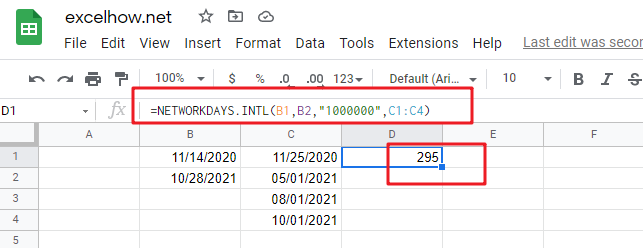The weekend string value “1000000” means that Monday is the weekend, and all other days are considered as working days.

This post will guide you how to use Google Sheets NETWORKDAYS function with syntax and examples.

## Description

The Google Sheets NETWORKDAYS function returns the number of net working days between tow given days.

The NETWORKDAYS function can be used to calculate the number of working days between dates in google sheets. And NETWORKDAYS will exclude weekends automatically. The purpose of this function is to get the number of working days between two dates and its returned values is a number that representing days.

The NETWORKDAYS function is a build-in function in Google Sheets and it is categorized as a Date function.

## Syntax

The syntax of the NETWORKDAYS function is as below:

=NETWORKDAYS (start_date, end_date, [holidays])

Where the NETWORKDAYS function argument is:

• Start_date -This is a required argument. The starting of date from which to calculate the number of working days.
• End_date – this is a required argument. The ending of date from which to calculate the number of working days.
• Holidays – this is an optional argument. A list of non-working days.

Note:

• NETWORKDAYS function only calculate all workdays, ignoring any time values.
• NETWORKDAYS function will exclude all Saturday and Sunday automatically.
• NETWORKDAYS function must be include both the starting date and ending date when calculating workdays.
• `Holidays` argument must be a date serial number values, or a date value returned by N function, or returned by DATE, DATEVALUE functions. It should be standard date values or date serial numbers.
• If you wish to use other days of week as the weekend, you can use the NETWORKDAYS.INTL function.

## Google Sheets NETWORKDAYS Function Examples

If you want to calculate the number of workdays between two dates in google sheets, you can use a formula based on the NETWORKDAYS function. And it will exclude all weekend days between the start dates and end dates. You need to specify two dates contain start date and end date so that you can get the number of workdays.

For example, you have two dates in range B1:B2, and you want to get the workdays between these two dates, you just need to use the following formula:

`=NETWORKDAYS(B1,B2)`

Type this formula in a blank Cell, and press Enter key in your keyboard.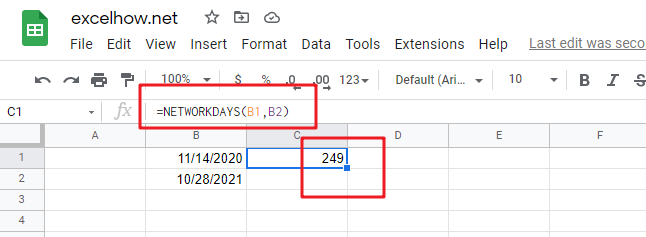The above formula contains the NETWORKDAYS function but does not take into account holidays, if you wish to take into account holidays( a range in C1:C4), just using the following formula:

`=NETWORKDAYS(B1,B2,C1:C4)`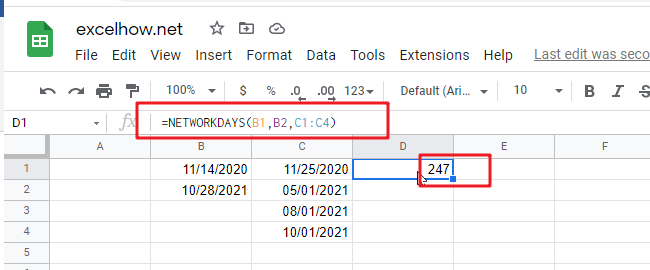This post will guide you how to use Google Sheets MONTH function with syntax and examples.

## Description

The Google Sheets MONTH function returns the month of a date represented by a serial number. And the month is an integer number from 1 to 12. And you can use the MONTH function to get a month component from a given date value. And you can feed the month number to another formula.

The MONTH function can be used to extract the month from a given date as a number between 1 to 12 in google sheets. The purpose of this function is to get MONTH as a number from a date and its returned values is a number between 1 and 12.

The MONTH function is a build-in function in Google Sheets and it is categorized as a Date function.

## Syntax

The syntax of the MONTH function is as below:

=MONTH (date)

Where the MONTH function argument is:

• Date -This is a required argument. A valid date value that contains the month component you want to extract. Dates should be entered by using the DATE function, or as results of other formulas or functions. For example, use DATE (2021,3,15) for the 15th day of March, 2021.

Note:

• You need to make sure that the Date argument is a cell reference that containing a date, or a function which returns a date value or a date serial number.
• If `Date` argument is not recognized, MONTH function will return #VALUE! Error message.
• If `Date` argument is supplied as a date number that is out of range, MONTH function will return #NUM! error message.
• You can use the MONTH function to extract a month component from a given date, or using this function to feed a month number into another function to create a more complex formula like the DATE function.
• Date argument can be supplied to the MONTH function as a date text string (e.g. “2021/11/13” or “Nov-11-2021”).  Or creating a date value with separate year, month, and day by using the DATE function.

## Google Sheets MONTH Function Examples

The below examples will show you how to use google sheets MONTH Function to return an integer number that representing the month component of a given date.

1# get month of the date in Cell A1, enter the following formula in Cell B1.

`=MONTH(A1)`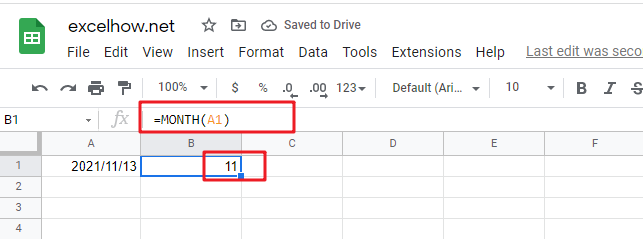2# convert Month name to number, type the following formula in Cell B1.

You should use the DATEVALUE function to convert a date stored as text to a serial number. Then feed the result into the MONTH function to extract a month number from date.

`=MONTH(DATEVALUE(A1 & "1"))`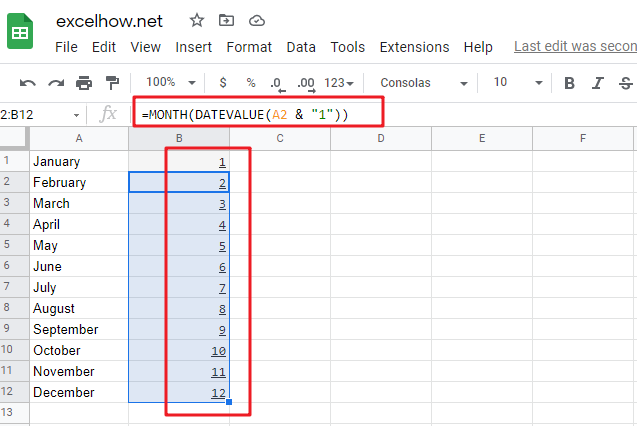This post will guide you how to use Google Sheets MINUTE function with syntax and examples.

## Description

The Google Sheets MINUTE returns the minutes of a time value. And the minutes is an integer number from 0 to 59. So you can use the MINUTE function to extract the minute component of a given time value.

The MINUTE function can be used to extract the minute component of a given time as a number between 0-59 in google sheets. The purpose of this function is to get minute as a number from a time and its returned values is a number between 0 and 59.

For example, for a valid time value 10:29 AM, the MINUTE function will return 29. So you can pass the returned result into another formula.

The MINUTE function is a build-in function in Google Sheets and it is categorized as a Date function.

## Syntax

The syntax of the MINUTE function is as below:

=MINUTE (time)

Where the MINUTE function arguments is:

• time -This is a required argument. A valid time value that contains the minute you want to extract. The times can be entered as a text strings within quotation marks (for example, “10:32 AM“), or typed as a decimal numbers, or the results of others formulas. It must be a cell reference that containing a date or time, or a function that returning a date/time type or a number.

Note:

• Time values are a portion of a date value and represented by a decimal number (for example, 12:00 PM is represented as 0.5, since it is half of a day).
• If the `time` is not a valid Excel time, it will return #VALUE! Error.
• `Time` argument can be supplied to the MINUTE function as a time text string or as a decimal numbers. If you wish to create a time value with separate hour, minute and second, you can use the TIME function.

## Google Sheets MINUTE Function Examples

The below examples will show you how to use google sheets MINUTE Function to return an integer number that representing the minute component of a given time.

#1 get minute portion of the time, enter the following formula in Cell C1.

`=MINUTE(B1)`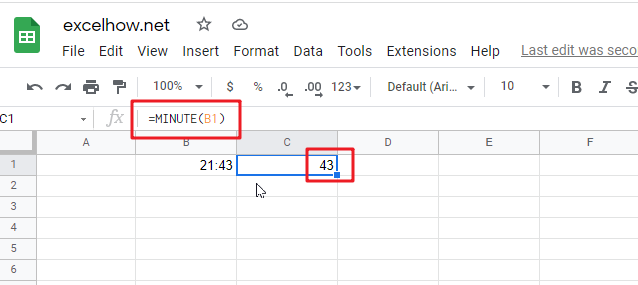This post will guide you how to use Google Sheets ISOWEEKNUM function with syntax and examples.

## Description

The Google Sheets ISOWEEKNUM returns the number of the ISO week of the year for a given date. ISO weeks begin on Monday and number 1 is the first week in that year.

The ISOWEEKNUM function can be used to get a week number from 1 to 54 that follows ISO standards in google sheets. The purpose of this function is to get ISO week number for a given data and its returned values is a number between 1 and 54.

The ISOWEEKNUM function is a build-in function in Google Sheets and it is categorized as a Date function.

## Syntax

The syntax of the ISOWEEKNUM function is as below:

=ISOWEEKNUM (date)

Where the ISOWEEKNUM function arguments is:

• Date -This is a required argument. The date for which to calculate the ISO week number. It must be a cell reference that containing a date value, number or a function that returning a date type in google sheets.

Note:

• You can use the DATE function to enter into a date value, or it will be return errors for a text values.
• If the `date` argument is not a valid date type, ISOWEEKNUM function returns the #VALUE! Error message.
• You need to know the WEEKNUM function will also start week number 1 on the first day of the year, then increment week numbers on Sundays.

## Google Sheets ISOWEEKNUM Function Examples

The below examples will show you how to use google sheets ISOWEEKNUM Function to get the ISO week number for a given date.

1# calculate the ISO week number for a given date in Cell B1, using the following formula:

`=ISOWEEKNUM(B1)`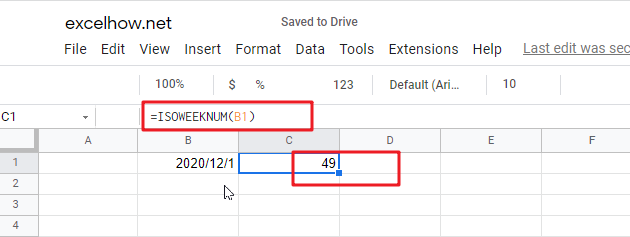2# calculate the ISO WEEK number of current date

If you want to get the ISO week number for the current date in google sheets, and you can use the ISOWEEKNUM formula in combination with the TODAY function, just using the following formula:

`=ISOWEEKNUM(TODAY())`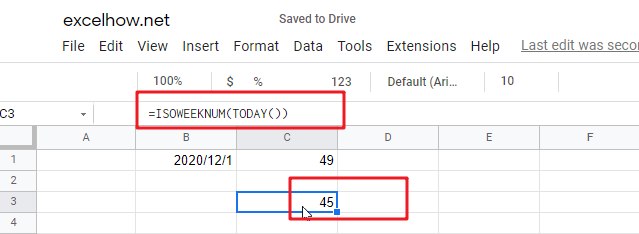3# calculate the ISO WEEK number with a date text string

You can enter a date text string into ISOWEEKNUM function instead of a cell reference that contain a date type value, just see the below formula:

`=ISOWEEKNUM("2010/8/20")`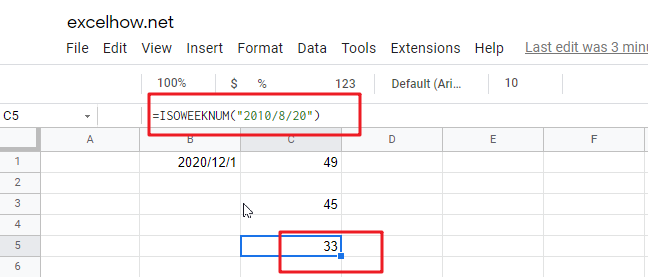This post will guide you how to use Google Sheets HOUR function with syntax and examples.

## Description

The Google Sheets HOUR returns the hour of a time value. Or returns an integer value that represent the hour component of a given time. And the return value is between 0-23.

For example, if you pass a time of 13:36 PM into HOUR function, and it will return 13. So you can use this function to extract the hour component from a time value.

The HOUR function can be used to get the hour component of a time as a number between 0-23 in google sheets. The purpose of this function is to get the hour as a number from a time and its returned values is a number between 0 and 23.

The HOUR function is a build-in function in Google Sheets and it is categorized as a Date function.

## Syntax

The syntax of the HOUR function is as below:

=HOUR (serial_number)

Where the HOUR function arguments is:

• Serial_text -This is a required argument. The time from which you want to extract the hour component. it may be typed as text string within quotation marks (for example, “13:52 PM”), or it can be a decimal numbers(for example, 0.5), or the result of a formula (for example, TIMEVALUE(“13.54PM”))

Note:

• Time values are a portion of a date value and represented by a decimal number (for example, 12:00 PM is represented as 0.5 because it is half of a day).
• A serial date is how the Microsoft Excel stores dates and times and it represents the number of days since 1900-01-01, so the January 1, 1900 date is serial number 1 by default.
• if the serial_number is not a valid Excel time, it will return #VALUE! error.

## Google Sheets HOUR Function Examples

The below examples will show you how to use google sheets HOUR Function to return the hour of a given time value.

1# get the hour component of 25% of 24 hours,, enter the following formula in Cell B1.

`=HOUR(0.25)`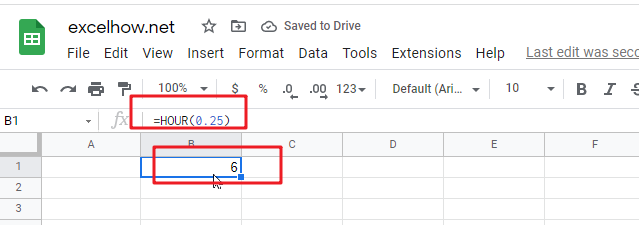2# get the hour portion of the date and time value, enter the following formula in Cell B2.

`=HOUR("11/10/2021 14:20")`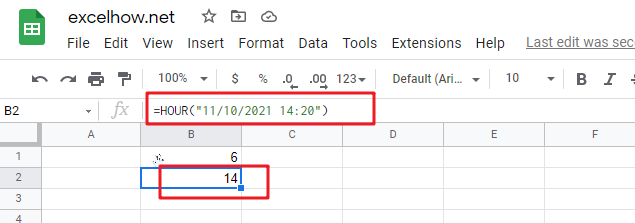3# Get the hour portion of the date with no time portion specified, enter the following formula in Cell B3.

`=HOUR("2021/11/10")`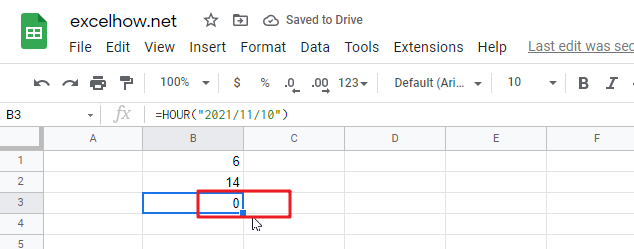so it will be considered 12:00AM or 0 hours.

This post will guide you how to use Google Sheets EOMONTH function with syntax and examples.

## Description

The Google Sheets EOMONTH returns a date representing the last day of the month, n months in the future or past.

The EOMONTH function can be used to get a date that representing the last day of a month which falls a specified number of months before or after date in google sheets. The purpose of this function is to get last day of month or n months in the future or past and its returned values is a last day of month date.

The EOMONTH function is a build-in function in Google Sheets and it is categorized as a Date function.

## Syntax

The syntax of the EOMONTH function is as below:

=EOMONTH (start_date, months)

Where the EOMONTH function arguments are:

• Start_date -This is a required argument. A date that represents the start date.
• months – This is a required argument. the number of months before or after start_date. if you provide a positive number for months, it represents the future date; if it is a negative number, then it represents a past date.

Notes:

• google sheets stores dates as a serial number, it indicated the number of days since January 1,1900. and the date January 1,1900 is serial number 1.
• if start_date is not a valid date, the EOMONTH function will return the #VALUE! error.
• if months is not an integer, it is truncated.
• If the start_date has a fractional time component, it will be removed.
• If the start_date argument contains a decimal value, it will be removed.
• if you want to change any date n months into the future or past date, you can use the EDATE function.

## Google Sheets EOMONTH Function Examples

The below examples will show you how to use google sheets EOMONTH Function to return the serial number.
1# set a positive number for months argument in the EOMONTH function

`=EOMONTH(B1,2)`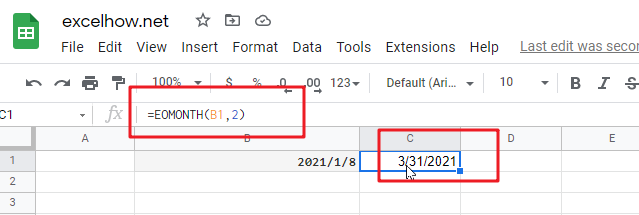2# set a negative number for months argument in the EOMONTH function, using the following formula:

`=EOMONTH(B1,-2)`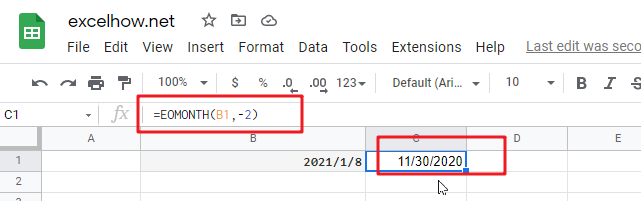3# set an invalid date for start_date argument in the EOMONTH function, type the following formula:

`=EOMONTH(B1,-2)`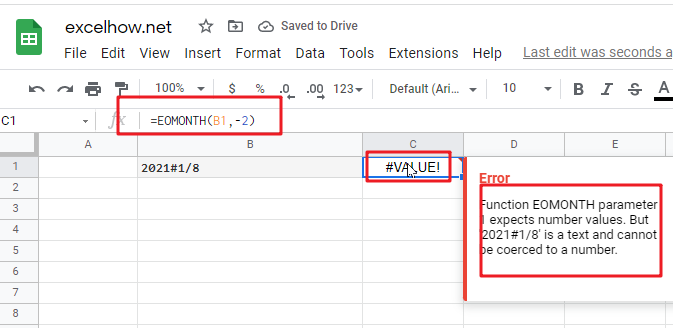4# set a value that is not an integer for months arguments, using the following formula:

`=EOMONTH(B1,2)`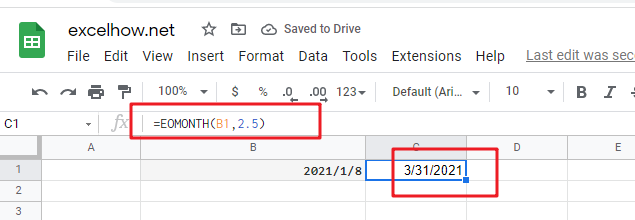This post will guide you how to use Google Sheets EDATE function with syntax and examples.

## Description

The Google Sheets EDATE returns a date that is a specified number of months before or after a specified date. You can use the EDATE function to calculate the expiration dates or maturity dates or due dates that fall on the same day of the month as the date of issue. or you can add a specified number of months to a date.

The EDATE function can be used to get a date a specified number of months before or after date in google sheets. The purpose of this function is to shift date a specified months in future or post and its returned values is a new date.

The EDATE function is a build-in function in Google Sheets and it is categorized as a Date function.

## Syntax

The syntax of the EDATE function is as below:

=EDATE (start_date, months)

Where the EDATE function arguments are:

• Start_date -This is a required argument. Adate that represents the start date.
• months – This is a required argument. the number of months before or after start_date. if you provide a positive number for months, it represents the future date; if it is a negative number, then it represents a past date.

Notes:

• google sheets stores dates as a serial number, it indicated the number of days since January 1,1900. and the date January 1,1900 is serial number 1.
• if `start_date` is not a valid date, the EDATE function will return the #VALUE! error.
• if months is not an integer, it is truncated.
• If the `start_date` has a fractional time component, it will be removed.
• If the `start_date` argument contains a decimal value, it will be removed.

## Google Sheets EDATE Function Examples

The below examples will show you how to use google sheets EDATE Function to return the serial number.
1# set a positive number for months argument in the EDATE function

`=EDATE(B1,2)`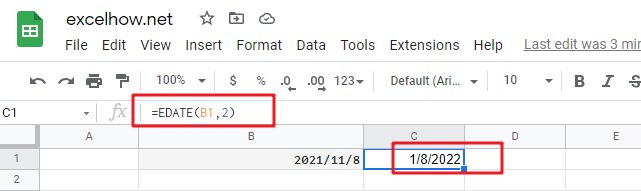2# set a negative number for months argument in the EDATE function, using the following formula:

`=EDATE(B2,-2)`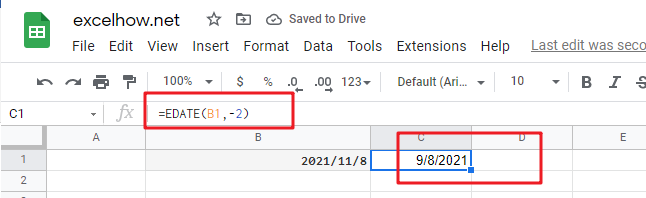3# set a invalid date for start_date argument in the EDATE function, type the following formula:

`=EDATE(B1,2)`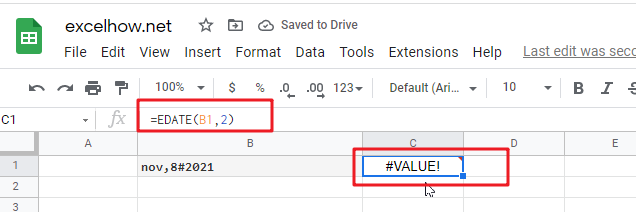4# set a value that is not an integer for months arguments, using the following formula:

`=EDATE(B4,2)`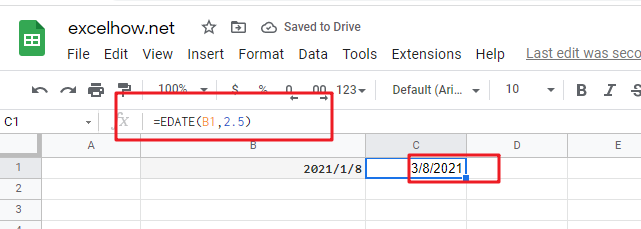This post will guide you how to use Google Sheets DAYS360 function with syntax and examples.

## Description

The Google Sheets DAYS360 will calculate the number of days between two dates based on a 360-day year.

The DAYS360 function can be used to get the number of days between two dates based on a 360-day year in google sheets. The purpose of this function is to get days between two dates in a 360-day year and its returned values is a number indicating days.

The DAYS360 function is a build-in function in Google Sheets and it is categorized as a Date function.

## Syntax

The syntax of the DAYS360 function is as below:

=DAYS360 (start_date, end_date,[method])

Where the DAYS360 function arguments are:

• Start_date and end_date -This is a required argument. The two dates to calculate the number of days.it must be a cell reference containing a date, or a function that returning a date type or a number.
• Method – This is an optional argument. It’s a Boolean value. If TRUE is set, the DAYS360 function will use the European method. Or it will use US method.

Note:

• The default value for Method argument is FALSE. It indicates the US method. when DAYS360 function is under the US method, the day of month of s`tart_date` is changed to 30th day of that month, if the `start_date` is the last day of the month. When the` end_date` is the last day of the month, and the `start date` is less than 30, `end_date` is changed to the first day of the month following `end_date`, otherwise the day of month of `end_date` is changed to 30.
• If DAY360 function is under European method, start and end dates equal to the 31st of a month has its day of month changed to 30.
• The DAYS360 function only works with whole numbers.
• If both dates are not valid, DAYS360 will return the #VALUE! Error.
• If both dates are out of range, DAYS360 will return the #NUM! error.

## Google Sheets DAYS360 Function Examples

The below examples will show you how to use google sheets DAYS360 Function to return the number of days between two dates based on a 360-day year.

#1 =DAYS360(B1,B2)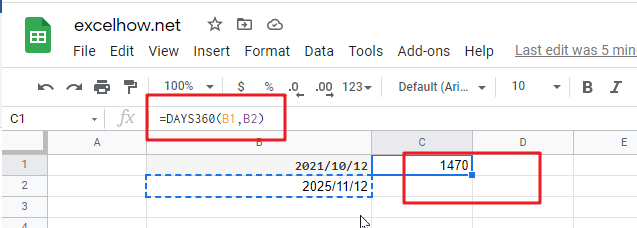Note: the above google sheets formula returns the number of days between 2021/10/12 and 2025/11/12, based on a 360-day year.

This post will guide you how to use Google Sheets DAYS function with syntax and examples.

## Description

The Google Sheets DAYS  returns the number of days between two dates.

The DAYS function can be used to get the number of days between two given dates in google sheets. The purpose of this function is to get days between dates and its returned values is a number indicating days.

The DAYS function is a build-in function in Google Sheets and it is categorized as a Date function.

## Syntax

The syntax of the DAYS function is as below:

= DAYS (end_date,start_date)

Where the DAYS function arguments is:

• End_date -This is a required argument. The end of the date
• Start_date – this is a required argument. The start of the date

Note:

• Both two date arguments must be a valid date value, or it will return the #VALUE! Error.
• The DAYS function only works with whole numbers.
• If end dates and start dates are reversed, the DAYS function will return a negative number.
• The DAYS function will return all days between two dates. If you want to calculate all working days between dates, you can use the NETWORKDAYS function in google sheets.

## Google Sheets DAYS Function Examples

The below examples will show you how to use google sheets DAYS Function to return the number of days between end_date and start_date.

#1 =DAYS (B2,B1)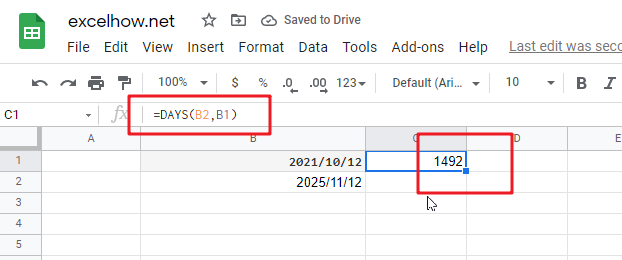Note: the above google sheets formula returns the number of days (1492) between the end date (2025/11/12) and start date (2021/10/12).